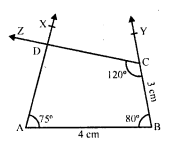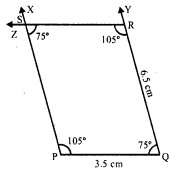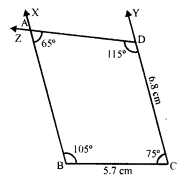# RD Sharma Class 8 Solutions Chapter 18 Practical Geometry Ex 18.5

In this chapter, we provide RD Sharma Class 8 Solutions Chapter 18 Practical Geometry Ex 18.5 for English medium students, Which will very helpful for every student in their exams. Students can download the latest RD Sharma Class 8 Solutions Chapter 18 Practical Geometry Ex 18.5 Maths pdf, free RD Sharma Class 8 Solutions Chapter 18 Practical Geometry Ex 18.5 book pdf download. Now you will get step by step solution to each question.

### RD Sharma Solutions for Class 8 Chapter 18 Practical Geometry Ex 18.5Download PDF

Question 1.
Construct a quadrilateral ABCD given that AB = 4 cm, BC = 3 cm, ∠A = 75°, ∠B = 80° and ∠C = 120°.
Solution:
Steps of construction :
(i) Draw a line segment AB = 4 cm.(ii) At A draw a ray AX making an angle of 75°.
(iii) At B draw another ray BY making an angle of 80° and cut off BC = 3 cm.
(iv) At C, draw another ray CZ making an angle of 120° which intersects AX at D.
Then ABCD is the required quadrilateral.

Question 2.
Construct a quadrilateral ABCD where AB = 5.5 cm, BC = 3.7 cm, ∠A = 60°, ∠B = 105° and ∠D = 90°.
Solution:
∠A = 60°, ∠B = 105° and ∠D = 90°
But ∠A + ∠B + ∠C + ∠D = 360° (Sum of angles of a quadrilateral)
⇒ 60° + 105° + ∠C + 90° = 360°
⇒ 255° + ∠C = 360°
⇒ ∠C = 360° – 255° = 105°
Steps of construction :
(i) Draw a line segment AB = 5.5 cm.
(ii) At A, draw a ray AX making an angle of(iii) At B, draw another ray BY making an angle of 105° and cut off BC = 3.7 cm.
(iv) At C, draw a ray CZ making an angle of 105° which intersects AX at D.
Then ABCD is the required quadrilateral.

Question 3.
Construct a quadrilateral PQRS where PQ = 3.5 cm, QR = 6.5 cm, ∠P = ∠R = 105° and ∠S = 75°.
Solution:
∠P = 105°, ∠R = 105° and ∠S = 75°
But ∠P + ∠Q + ∠R + ∠S = 360° (Sum of angles of a quadrilateral)
⇒ 105° + ∠Q + 105° + 75° = 360°
⇒ 285° + ∠Q = 360°
⇒ ∠Q = 360° – 285° = 75°
Steps of construction :
(i) Draw a line segment PQ = 3.5 cm.(ii) At P, draw a ray PX making an angle of 105°.
(iii) At Q, draw another ray QY, making an angle of 75° and cut off QR = 6.5 cm.
(iv) At R, draw a ray RZ making an angle of 105° which intersects PX at S.
Then PQRS is the required quadrilateral.

Question 4.
Construct a quadrilateral ABCD when BC = 5.5 cm, CD = 4.1 cm, ∠A = 70°, ∠B = 110° and ∠D = 85°.
Solution:
∠A = 70°, ∠B = 110°, ∠D = 85°
But ∠A + ∠B + ∠C + ∠D = 360° (Sum of angles of a quadrilateral)
⇒ 70° + 110° + ∠C + 85° = 360°
⇒ 265° + ∠C = 360°
⇒ ∠C = 360° – 265° = 95°
Steps of construction:
(i) Draw a line segment BC = 5.5 cm.
(ii) At B, draw a ray BX making an angle of 110°.
(iii) At C, draw another ray CY making an angle of 95° and cut off CD = 4.1 cm.
(iv) At D, draw a ray DZ making an angle of 85° which intersects BX at A.Then ABCD is the required quadrilateral.

Question 5.
Construct a quadrilateral ABCD, where ∠A = 65°, ∠B = 105°, ∠C = 75°, BC = 5.7 cm and CD = 6.8 cm.
Solution:
∠A = 65°, ∠B = 105°, ∠C = 75°
But ∠A + ∠B + ∠C + ∠D = 360° (Sum of angles of a quadrilateral)
⇒ 65° + 105° + 75° + ∠D = 360°
⇒ 245° + ∠D = 360°
⇒ ∠D = 360° – 245° = 115°
Steps of construction:
(i) Draw a line segment BC = 5.7 cm.
(ii) At B, draw a ray BX making an angle of(iii) At C draw a another ray CY making an angle of 75° and cut off CD = 6.8 cm.
(iv) At D, draw a ray DZ making an angle of 115° which intersects BX at A.
Then ABCD is the required quadrilateral.

Question 6.
Construct a quadrilateral PQRS in which PQ = 4 cm, QR = 5 cm, ∠P = 50°, ∠Q = 110° and ∠R = 70°.
Solution:
Steps of construction :
(i) Draw a line segment PQ = 4 cm.
(ii) At P, draw a ray PX making an angle of 50°.
(iii) At Q, draw another ray QY making an angle of 110° and cut off QR = 5 cm.
(iv) At R, draw a ray RZ making an angle of 70° which intersects PX at S.Then PQRS is the required quadrilateral.

All Chapter RD Sharma Solutions For Class 8 Maths

—————————————————————————–

All Subject NCERT Exemplar Problems Solutions For Class 8

All Subject NCERT Solutions For Class 8

*************************************************

I think you got complete solutions for this chapter. If You have any queries regarding this chapter, please comment on the below section our subject teacher will answer you. We tried our best to give complete solutions so you got good marks in your exam.

If these solutions have helped you, you can also share rdsharmasolutions.in to your friends.